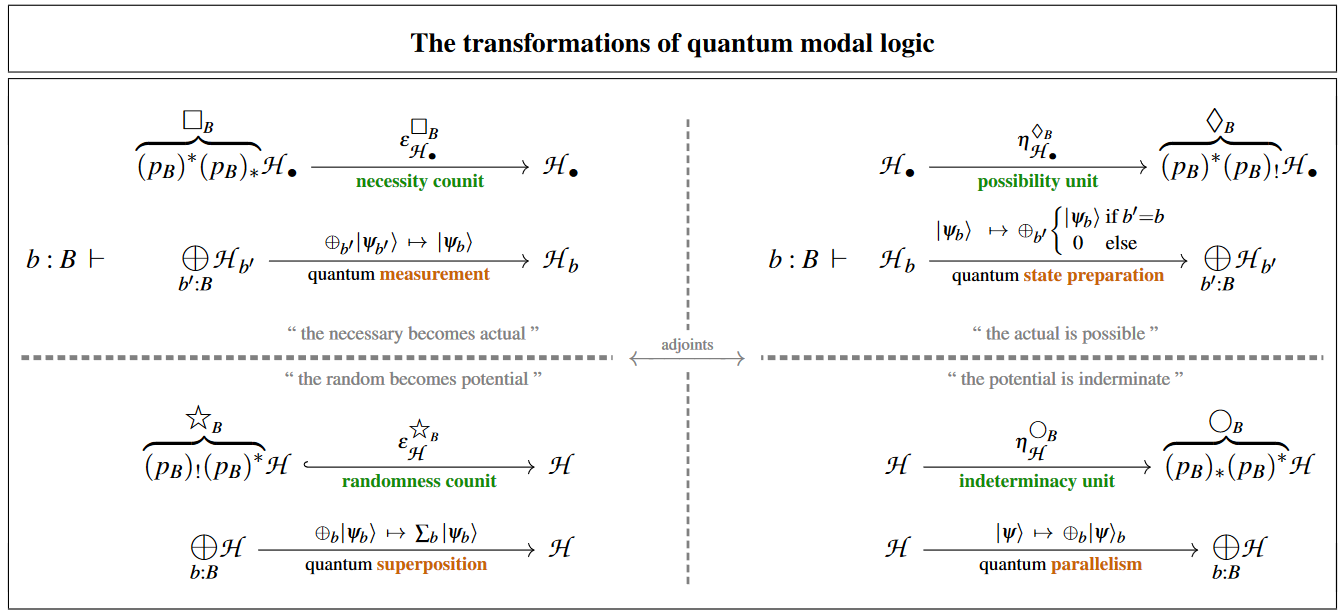Contents

# Contents

## Idea

In quantum theory the state of a physical system is given by a vector in a complex vector space (usually a Hilbert space). This means that given any two states $\psi_1$ and $\psi_2$, their sum, the vector $\psi_1 + \psi_2$, also represents a state. This is called the quantum superposition of the two states.

If these quantum states are thought of as wavefunctions solving a linear differential equation such as the Schroedinger equation, Dirac equation, Klein-Gordon equation or Tomonaga-Schwinger equation, their quantum superposition is an example of the general principle of superposition of solutions to linear differential equations.

The concept of quantum superposition is at the heart of what makes quantum physics peculiar, notably via the phenomenon of entanglement.

The various fundamental quantum physics phenomena at play here may be identified with four modal-units of quantum modal logic (as discussed at quantum circuits via dependent linear types):quantum measurementquantum state preparation
quantum superpositionquantum parallelism